# The Programmatic Side of Mathematica II

Since the last time I posted, I found a better way to do 100 dice rolls: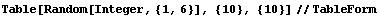This gives 100 dice rolls in a tabulated format in a much simpler syntax. You can also make a “die” out of complex numbers, but it is a little more of an issue to truncate: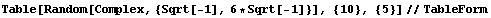… which gives output like: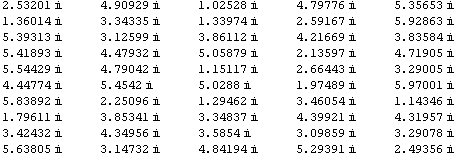Strictly speaking, these are all a kind of complex number called a pure imaginary number, but I guess I can’t split hairs.

You can create a list of divisors of integers like 28, like so:

```Divisors
{1, 2, 4, 7, 14, 28}```

28 is a called a perfect number, because like 6, the number can be created by the sum of its proper factors. A “proper factor” of a number k does not include k itself, so if you add the numbers generated in the above list, you will obtain 2k, or 56.

So to get the correct result, you can make your own function that can re-generate the list with the last element truncated. The Drop[] function can do this: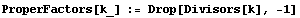I have declared a small function called ProperFactors, and the underscore after the k indicates that it is a placeholder for a parameter. When you run this, it returns nothing, but of course all you really did was declare a function. The Drop[] function drops elements from the list generated by the Divisors[] function, beginning at the last element (the reason there is a “-1” passed to Drop[]. In the list generated before, the last element was the highest factor of 28, which was 28 itself. The remaining elements in the list are the proper factors.

So, the call:

`ProperFactors`

generates the list:

`{1, 2, 4, 7, 14}`

which are the proper factors of 28. Let’s programmatically check to see if the sum of the proper factors add to 28. Of course, checking by adding the numbers up yourself will tell you this: 1 + 2 + 4 + 7 + 14 = 28. But making a function do the work will be beneficial if the factors become very large. So, we can make use of the Apply[] function:

`Apply[Plus, {1,2,3,4,5}]`

This returns:

`15`

or the sum of 1 + 2 + 3 + 4  + 5. It takes a list of numbers (an actual array), and treats them as though each element were parameters passed into the Plus[] function. I could get the same thing with:

`Plus[1, 2, 3, 4, 5]`
`15`

The curly braces aren’t needed, since Plus[] expects a list of arbitrary length, but not an array. Since ProperFactors[] generates an array, I need to convert the array into a list to be operated on by Plus[]. This is the reason for the Apply[] function.

List[] is a function which generates an array:

`List[1,2,3,4,5]`
`{1, 2, 3, 4, 5}`

And simply invoking the literal array {1, 2, 3, 4, 5} does the same thing as List[]. And you can assign the array to a variable.

`s := List[2, 3, 4, 5, 6]`

does the same thing as

`s:= {2, 3, 4, 5, 6}`

so that

`Apply[Plus, s]`

converts the array s to a list of parameters passed to Plus. The result is:

`20`

Now, let’s go back to our perfect number checking function. Let’s call it PerfectCheck[]:

`PerfectCheck[k_] := Apply[Plus, ProperFactors[k]] == k`

What does “Apply[Plus, ProperFactors[k]]” do? Let’s invoke that by itself in Mathematica to see, using k = 28:

`Apply[Plus, ProperFactors]`
`28`

so, it adds up the proper factors of 28. To make sure, let’s use a non-perfect composite number:

`Apply[Plus, ProperFactors]`
`117`

To check this, go further back and list the proper factors of 100 themselves: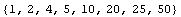You will see when adding by hand that these add to 117.

Thus, a PerfectCheck would be false since 117 <> 100.

`PerfectCheck`
`false`
`PerfectCheck`
`true`
`PerfectCheck`
`true`
`PerfectCheck`
`false`

Checking numbers in the range 1 to n can be done using Select[]:

`perfect[n_] := Select[Range[n], PerfectCheck]`

So that:

`perfect`

yields:

`{6, 28, 496, 8128}`

I was trying to find a fifth perfect number, but it’s difficult. I even cranked up the call to perfect but no luck, with Mathematica chugging away for about 10 minutes. But I was able to find information through Google more quickly. According to The Math Forum, they say that the fifth one was still some ways off: 33,550,336.

A sixth number may exist, but as you can see the trend seems to indicate that they are pretty sparse on the number line. The Math forum hinted that these perfect numbers obey a number pattern according to the formula: (2n)(2n+1 – 1). This isn’t really reliable; that is to say that the value of integer used for n will not generate the nth perfect number necessarily. This seems to generate a perfect number so long as 2n+1 – 1 equals the value of a Mersenne Prime. A Mersenne Prime is a prime number that is one less than some power of 2, such as the number 7, which is 23 – 1. When finding the “highest known prime number” these days, mathematicians look for Mersenne Primes.

Using a command like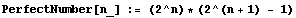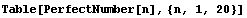can fool you into thinking you have just printed out the first 20 perfect numbers, but you would be wrong. The list it gives out: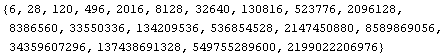has numbers, such as 120, 496, and 2016, that are not perfect. You still need to check each one with our PerfectCheck[] function (either that, or fish out the numbers with Mersenne prime factors among the lot).

You would have to keep hunting until you find 8,589,869,056 as being the sixth perfect number, closer to the end of the list. Then the seventh one is two more over: 137,438,691,328.

But, of course we have a computer, so life gets happy once again for the slack among us. We can mash up the Table[] call above, and Select[] numbers that are perfect with PerfectCheck: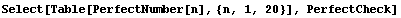which generates the array: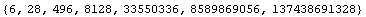and all of these are perfect numbers. I can go even more nutzo and increase the upper limit to 100: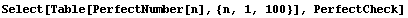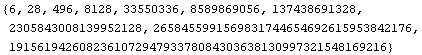… thereby generating the first 10 perfect numbers.

There are to date only 47 known Mersenne primes according to Wikipedia. Therefore, there are only 47  known perfect numbers, assuming all perfect numbers are even.